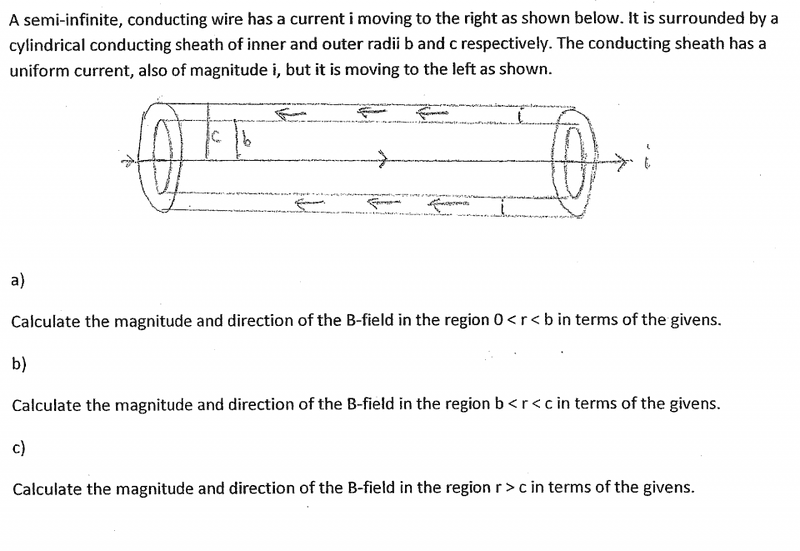# Magnetic Field in a Semi-Infinite Wire

## Homework Statement## Homework Equations

B = μi / 4πR is the equation for a semi-infinite straight wire

## The Attempt at a Solution

I know that for each situation in a,b, and c I would use the equation I listed above, but I am not sure what I would plug in for R for each situation.

haruspex
Homework Helper
Gold Member

Thanks, but I know which equations to use, I just don't know how to apply them. I don't know what to plug in for r in each situation.

TSny
Homework Helper
Gold Member
The problem is not clear concerning the location of the points where you are to find B. For a fixed value of r, the field will vary as you move parallel to the axis of the cylinder. I guess you are to assume that you are looking for B only at points in a plane that is perpendicular to the axis and located at the end of the cylinder.

Likewise, the formula you gave for a semi-infinite wire is only valid for points in a plane oriented perpendicular to the wire and located at the end of the wire.

To work this problem you need to understand how to obtain the formula you gave for the semi-infinite wire. The same reasoning can be used to help solve the cylinder problem.

haruspex